# Consider the given function and the given interval.f(x) = 18 sin(x) – 9 sin(2x), [0, x](a) Find the average value fave of f on the given interval.fave%3D(b) Find c such that fave = f(c). (Round your answers to three decimal places.)(smaller value)(larger value)

Question
40 views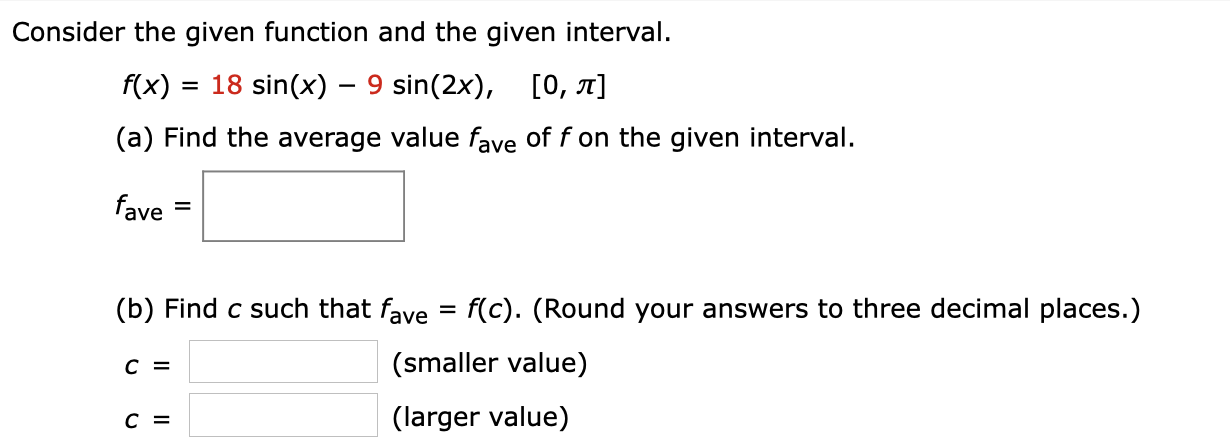help_outlineImage TranscriptioncloseConsider the given function and the given interval. f(x) = 18 sin(x) – 9 sin(2x), [0, x] (a) Find the average value fave of f on the given interval. fave %3D (b) Find c such that fave = f(c). (Round your answers to three decimal places.) (smaller value) (larger value) fullscreen
check_circle

star
star
star
star
star
1 Rating
Step 1

Average value fave of f(x) on the given interval [a, b] is given by -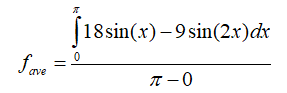Difference rule of integration is given by –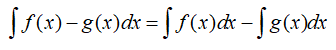Step 2

Given –

a)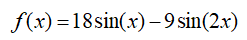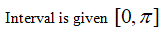Substituting given values of f(x), a and b in the formula.Using a difference rule of integration can be rewritten as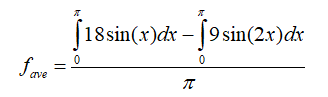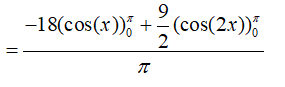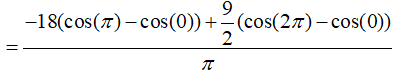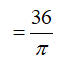Step 3

b)               We have to find c such that favg =f(c)

Substituting x = c in f(x) we will get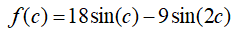So according to the question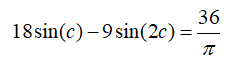Using graphing calculator we can find two values of c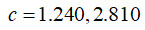...

### Want to see the full answer?

See Solution

#### Want to see this answer and more?

Solutions are written by subject experts who are available 24/7. Questions are typically answered within 1 hour.*

See Solution
*Response times may vary by subject and question.
Tagged in